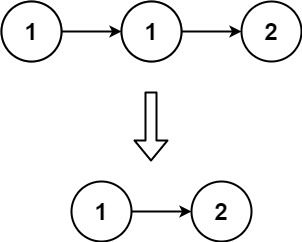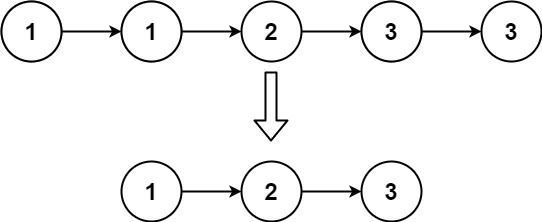## Algorithm

Problem Name: 83. Remove Duplicates from Sorted List

Given the `head` of a sorted linked list, delete all duplicates such that each element appears only once. Return the linked list sorted as well.

Example 1:```Input: head = [1,1,2]
Output: [1,2]
```

Example 2:```Input: head = [1,1,2,3,3]
Output: [1,2,3]
```

Constraints:

• The number of nodes in the list is in the range `[0, 300]`.
• `-100 <= Node.val <= 100`
• The list is guaranteed to be sorted in ascending order.

## Code Examples

### #1 Code Example with C Programming

```Code - C Programming```

``````
struct ListNode* deleteDuplicates(struct ListNode* head) {
struct ListNode *prev, *p;

p = prev->next;

while (p) {
if (prev->val != p->val) {
prev->next = p;
prev = p;

p = p->next;
}
prev->next = NULL;

}
``````
Copy The Code &

Input

cmd

Output

cmd
[1,2]

### #2 Code Example with C++ Programming

```Code - C++ Programming```

``````
// Recursive
class Solution {
public:
}
};

// Non-recursive
class Solution {
public:
while(cur){
pre->val == cur->val ? pre->next = cur->next : pre = cur;
cur = cur->next;
}
}
};
``````
Copy The Code &

Input

cmd

Output

cmd
[1,2]

### #3 Code Example with Java Programming

```Code - Java Programming```

``````
class Solution {
while (curr != null) {
while (curr.next != null && curr.next.val == curr.val) {
curr.next = curr.next.next;
}
curr = curr.next;
}
}
}
``````
Copy The Code &

Input

cmd

Output

cmd
[1,2,3]

### #4 Code Example with Javascript Programming

```Code - Javascript Programming```

``````
while (current !== null && current.next !== null) {
if (current.val === current.next.val) {
current.next = current.next.next;
} else {
current = current.next;
}
}
};
``````
Copy The Code &

Input

cmd

Output

cmd
[1,2,3]

### #5 Code Example with Python Programming

```Code - Python Programming```

``````
class Solution:
return root
``````
Copy The Code &

Input

cmd

Output

cmd
[1,2]

### #6 Code Example with C# Programming

```Code - C# Programming```

``````
namespace LeetCode
{
public class _083_RemoveDuplicatesFromSortedList
{
{
if (head == null) { return null; }

while (p.next != null)
{
if (p.val == p.next.val)
p.next = p.next.next;
else
p = p.next;
}

}
}
}
``````
Copy The Code &

Input

cmd